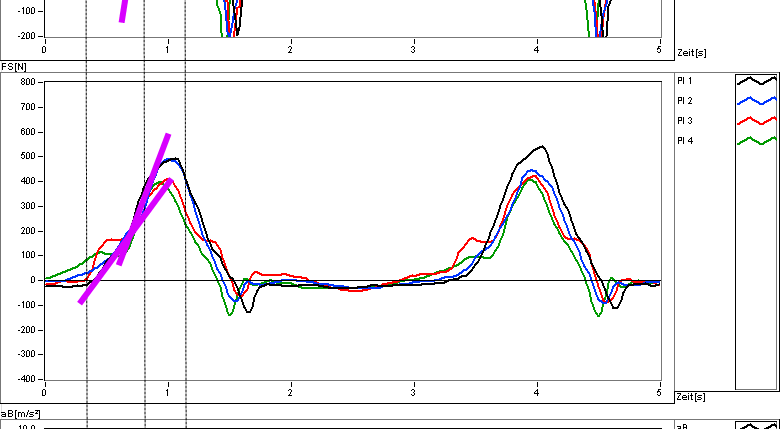# Understanding Force Curves and Boat Acceleration

In this post I want to take a look at the relation between “force curves” and boat acceleration. I will also touch on the topic of the mystical drive hump and demonstrate where it comes from.

To begin with, let us freshen up on some basic relations between forces, acceleration and speed.

1. When a force is applied to an object, the object changes it’s velocity.
2. Velocity is the speed that an object is moving with into a certain direction
3. The temporal rate of change in velocity is called acceleration and is directly proportional to the force applied divided by the mass of that object.

These relations are covered by the first and second Newton’s laws of motions and summed up in the equation F=m*a.

Forces and velocity are both vector quantities, which mean they have magnitude and direction. The force that determines the change in velocity is called the net force,  and is the result of adding all the individual force vectors that act on the object.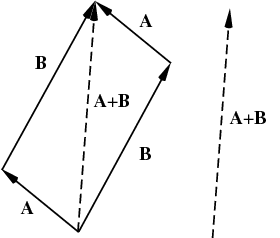Visualized, this means that the net force vector is the vector that we get by chaining all individual force vectors (e.g. A, B) and drawing a vector from start to finish (A+B).

## Principal Forces in Rowing

When we want to determine how a rowing  boat is moved forward, we need to look at the forces that are applied to the boat. In a simplified model, there are three principal forces acting on the rowing boat: gate force and stretcher force and drag force.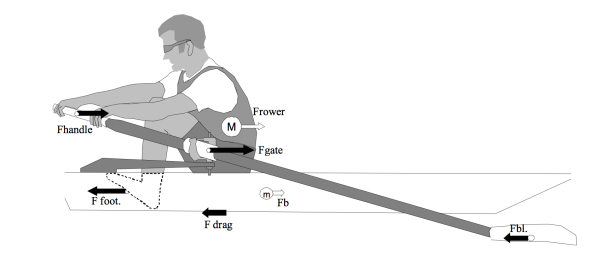Main forces on a rowing boat, source V. Kleshnev: Boat acceleration, temporal structure of the stroke cycle, and effectiveness in rowing, Proceedings of the Institution of Mechanical Engineers 2010

For the question of boat speed, we can disregard forces that do not directly on the boat such as the handle force or blade force.

Gate force is directed in the direction of travel, while stretcher and drag force work against it. Within reasonably limits, we can accept drag force as a given function of boat speed (drag is proportional to the cube of speed) and outside conditions (wind).

Because stretcher force works against gate force, we do always need to keep in mind how these two forces interact to create the net boat force which determines acceleration and thus boat speed. It is very important to not loose track of this big picture” when focusing on optimizing e.g. peak force. Worst case, a crew trying to exert a higher gate force while simultaneously increasing stretcher force will only have a higher cardiovascular load, for no gain in boat speed.

## Boat Acceleration is Net Boat Force

So now that we know that it is important to focus on improving the net boat force to achieve a higher boat speed, the next question is how do we measure this? If you could follow me until this point, I hope the answer is obvious. Since boat acceleration is directly proportional to the net boar force, it is the perfect proxy for somehow measuring or calculating the net boat force.

To illustrate the point I made above, let us look at the structure of gate force, stretcher force and boat. In the diagrams below, the first chart shows gate force, the second stretcher force and the third diagram boat acceleration over time.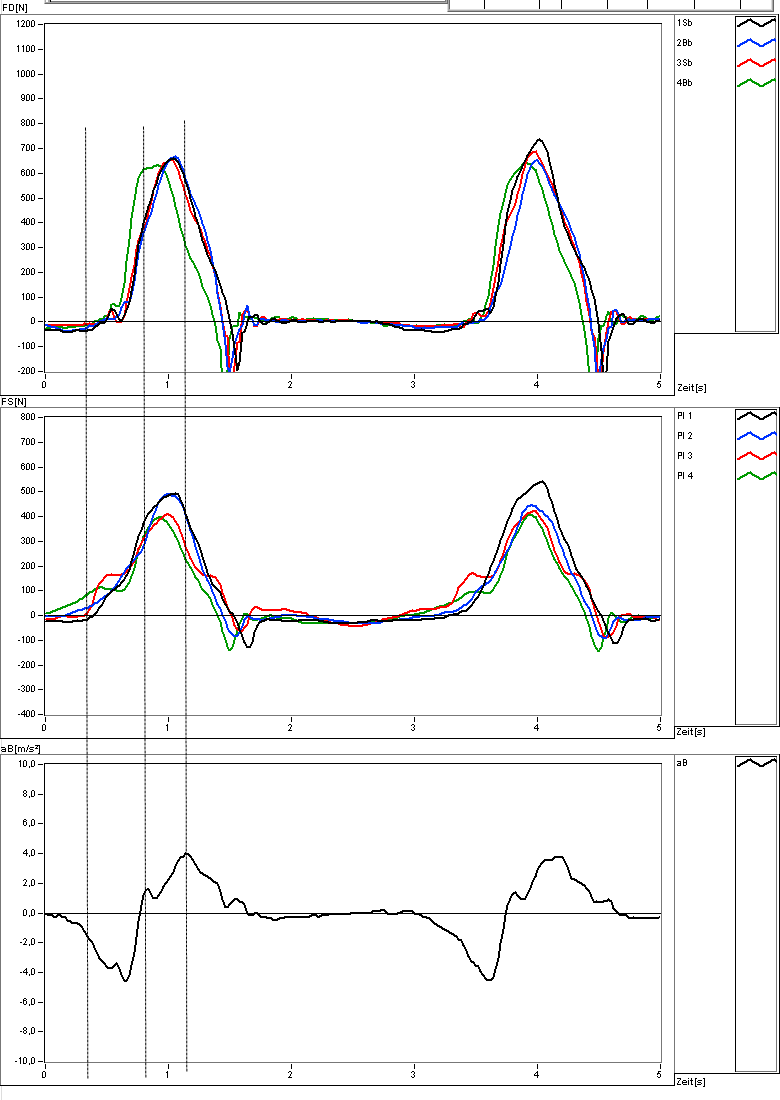I have placed three perfectly vertical lines in the graphs to make it easier to align the measurements for comparison. Let us disregard the obvious synchronicity issues in the crew for a second and look at the first 0.5s. We can see that stretcher force start to rise while gate force remains at zero, resulting in a deceleration of the boat. At about 0.7s, before the second vertical line, the boat has experiences zero acceleration, even though stretcher and handle force are close to 50% of their respective maximum. At this point, the crew performs a lot of biological work to create the force, yet nothing happens. Because the direction of stretcher and gate force are opposite, they cancel each other out.

Next, we see a positive acceleration of the boat with the gate force exceeding stretcher force. However, at about 0.8s, shortly after the second vertical line the boat acceleration = net boat force show a “dip”. Where does this dip come from?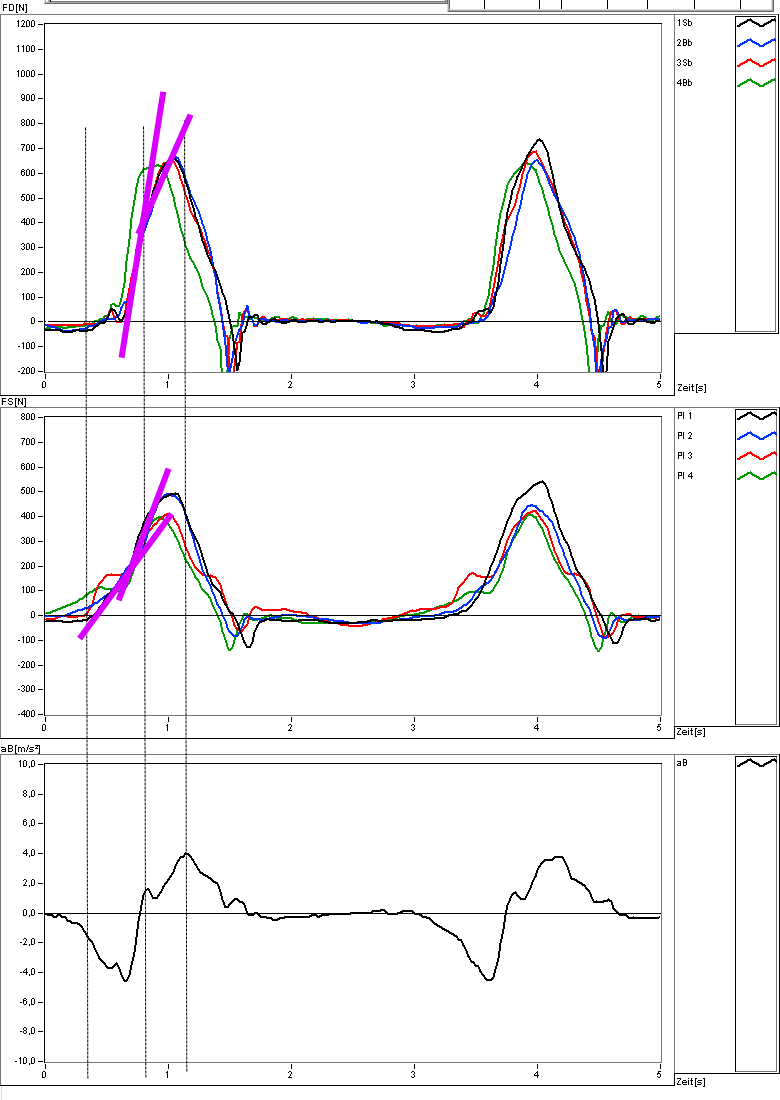I have marked the critical parts in the force curves with a thick purple line. Because they cover all graphs, they are a good approximation for the “crew total” force. Looking at the gate force, we see that it’s slope decreases at this critical point while the slope of the stretcher force increases. As a result, the sum of stretcher and gate force decreases for a short while. This is what creates the drive hump. Why this appears to be a biomechanic necessity is an interesting question for another article. What this crew did right however was to maintain a significant positive boat acceleration even when boat acceleration temporarily dipped.

## Working with Boat Acceleration

With Rowing in Motion you can easily measure boat acceleration and thus observe net boat force. The simplest option to get started is to use our mobile App running on a smartphone attached to the boat, which will use the builtin accelerometers to capture boat acceleration and display it in a live graph along with speed, stroke rate and many other interesting this.If you want to learn more about effectively using boat acceleration and what to look for, have a look at our guide for analyzing boat acceleration to get faster.

•It is the force on the pin that moves a boat. How if we get a hydraulic jack and place one end on the stretcher and the other end at the pin and we apply force. The boat does not move however much pressure we apply.

Thus we must apply the force in relation to an object external to the boat . Thus if we push the stretcher with a pole from a bank the boat will move backwards. Similarly if we apply force to the pin with an oar with its blade in the water the boat will move.

If we regard the blade as the fulcrum., then the force on the handle x length of oar (say 12 feet) = force on the pin x length of oar from collar to blade (say 8 feet). Thus the force on the oar handle should be two thirds of that on the pin.

The force on the stretcher should be irrelevant as it is not connect to an outside object as the pin is with the oar. It is only relevant for Newtons third law when the body mass is freewheeling towards the bows from bum shoving as it connects the boat to the body mass.

Stretcher force is useful to measure other factors such as “Mass Suspension”, bum shoving, body stress and for relation to boat check, and timing.

I find it hard to accept that a boat moves only when gate force (pin force) exceeds stretcher force. A boat moves (accelerates) when the gate force exceeds the de-accelerating force. Gate force is only created when there is a fulcrum, i.e. the blade is locked on in the water. The delay, or dip in acceleration is caused by two factors, first due to Newtons third law when the body mass is thrown up to the bows and secondly by the delay in locking on and finding a fulcrum. That it happens when the pin force exceeds the stretcher force is just a co-incidence or effect and not a cause, except to say that the de-accelaration (braking on the slide) of the body mass towards the stern and the acceleration towards the bows does result in forces on the stretcher. However once teh blade is buried the stretcher forces are irrelevant save that they are proportional (if not equal to) the forces on the oar handle.

•Great comment. I believe there’s a mismatch between what we’re talking about. The article looks at the acceleration of the boat. You’re talking about the acceleration of the rower/boat system.

When we’re talking about the acceleration of the rower/boat system as a whole, your line of thought is correct. In either case, the boat or the rower+boat system can only accelerate when they exchange force with another system.

Since actio=reactio, your hydraulic jack scenario would indeed not move the boat – because Fstrecther=Fpin (and vectors are opposite).

The actual equation for boat (and only boat) acceleration would be like this (I excluded some effects in the article to keep it simple):
a = Ftotal / m = (Fpin-Fstrechter-Fdrag-Fothers), where Fothers contains wind drag, sliding seat friction etc.)

The stretcher force vs. pin force topic is extremely important when you want to consider when an athlete generates _boat_ propulsion. I believe stretcher force is indeed irrelevant if we are interested in _rower+boat system_ propulsion, so you have a very good point there.

Since we’re looking into _boat_ acceleration, the dip is indeed caused only by stretcher vs pin force I would say. Pin force can indeed only exist if there’s a similar blade force (actio = reactio), so your “locking on” description is spot on.

Efficiently transferring stretcher force to the oar handle is an efficiency aspect as some of that force may disappear into internal friction into the athletes non-rigid body (I’d need to think that through properly though).

This is a very good discussion, a big thanks for your comments!